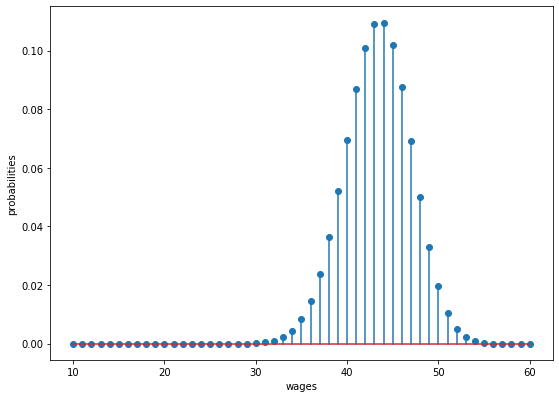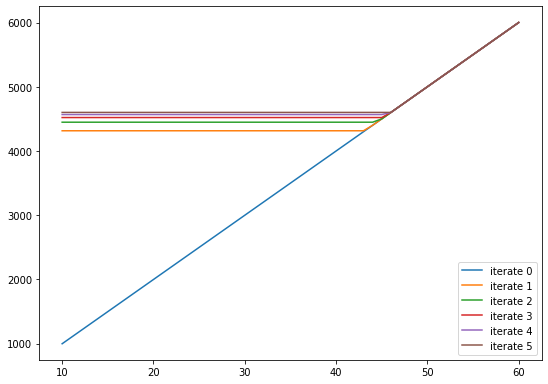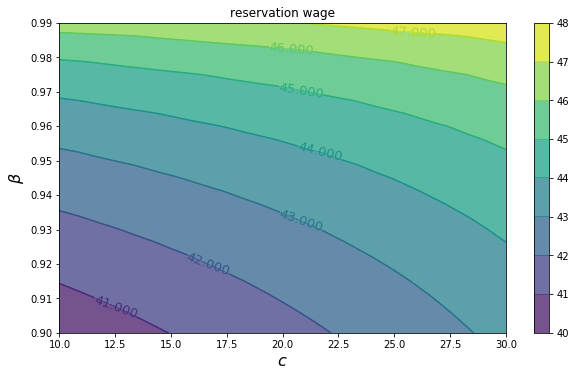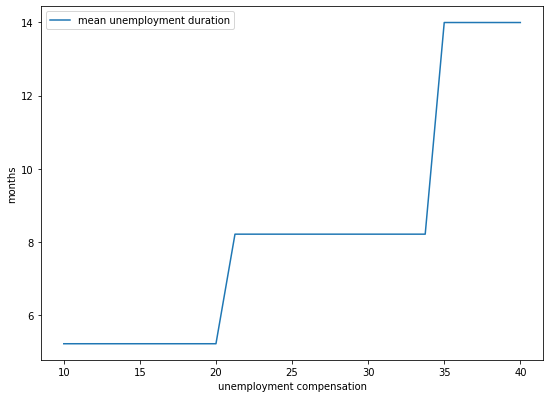How to read this lecture...

Code should execute sequentially if run in a Jupyter notebook

# Job Search I: The McCall Search Model¶

## Contents¶

“Questioning a McCall worker is like having a conversation with an out-of-work friend: ‘Maybe you are setting your sights too high’, or ‘Why did you quit your old job before you had a new one lined up?’ This is real social science: an attempt to model, to understand, human behavior by visualizing the situation people find themselves in, the options they face and the pros and cons as they themselves see them.” – Robert E. Lucas, Jr.

In addition to what’s in Anaconda, this lecture will need the following libraries:

In :
!pip install --upgrade quantecon


## Overview¶

The McCall search model [McC70] helped transform economists’ way of thinking about labor markets.

To clarify vague notions such as “involuntary” unemployment, McCall modeled the decision problem of unemployed agents directly, in terms of factors such as

• current and likely future wages
• impatience
• unemployment compensation

To solve the decision problem he used dynamic programming.

Here we set up McCall’s model and adopt the same solution method.

As we’ll see, McCall’s model is not only interesting in its own right but also an excellent vehicle for learning dynamic programming.

In :
import numpy as np
from numba import jit
import matplotlib.pyplot as plt
import quantecon as qe
from quantecon.distributions import BetaBinomial


## The McCall Model¶

An unemployed worker receives in each period a job offer at wage $W_t$.

At time $t$, our worker has two choices:

1. Accept the offer and work permanently at constant wage $W_t$.
2. Reject the offer, receive unemployment compensation $c$, and reconsider next period.

The wage sequence is assumed to be IID with probability mass function $\phi$.

Thus $\phi (w)$ is the probability of observing wage offer $w$ in the set $w_1, \ldots, w_n$.

The worker is infinitely lived and aims to maximize the expected discounted sum of earnings

$$\mathbb{E} \sum_{t=0}^{\infty} \beta^t Y_t$$

The constant $\beta$ lies in $(0, 1)$ and is called a discount factor.

The smaller is $\beta$, the more the worker discounts future utility relative to current utility.

The variable $Y_t$ is income, equal to

• his wage $W_t$ when employed
• unemployment compensation $c$ when unemployed

The worker faces a trade-off:

• Waiting too long for a good offer is costly, since the future is discounted.
• Accepting too early is costly, since better offers might arrive in the future.

To decide optimally in the face of this trade-off, we use dynamic programming.

Dynamic programming can be thought of as a two-step procedure that

1. first assigns values to “states” and
2. then deduces optimal actions given those values

We’ll go through these steps in turn.

### The Value Function¶

In order to optimally trade-off current and future rewards, we need to think about two things:

1. the current payoffs we get from different choices
2. the different states that those choices will lead to in next period (in this case, either employment or unemployment)

To weigh these two aspects of the decision problem, we need to assign values to states.

To this end, let $v^*(w)$ be the total lifetime value accruing to an unemployed worker who enters the current period unemployed but with wage offer $w$ in hand.

More precisely, $v^*(w)$ denotes the value of the objective function (1) when an agent in this situation makes optimal decisions now and at all future points in time.

Of course $v^*(w)$ is not trivial to calculate because we don’t yet know what decisions are optimal and what aren’t!

But think of $v^*$ as a function that assigns to each possible wage $w$ the maximal lifetime value that can be obtained with that offer in hand.

A crucial observation is that this function $v^*$ must satisfy the recursion

$$v^*(w) = \max \left\{ \frac{w}{1 - \beta}, \, c + \beta \sum_{w'} v^*(w') \phi (w') \right\} \tag{1}$$

for every possible $w$ in $w_1, \ldots, w_n$.

This important equation is a version of the Bellman equation, which is ubiquitous in economic dynamics and other fields involving planning over time.

The intuition behind it is as follows:

• the first term inside the max operation is the lifetime payoff from accepting current offer $w$, since
$$w + \beta w + \beta^2 w + \cdots = \frac{w}{1 - \beta}$$
• the second term inside the max operation is the continuation value, which is the lifetime payoff from rejecting the current offer and then behaving optimally in all subsequent periods

If we optimize and pick the best of these two options, we obtain maximal lifetime value from today, given current offer $w$.

But this is precisely $v^*(w)$, which is the l.h.s. of (1).

### The Optimal Policy¶

Suppose for now that we are able to solve (1) for the unknown function $v^*$.

Once we have this function in hand we can behave optimally (i.e., make the right choice between accept and reject).

All we have to do is select the maximal choice on the r.h.s. of (1).

The optimal action is best thought of as a policy, which is, in general, a map from states to actions.

In our case, the state is the current wage offer $w$.

Given any $w$, we can read off the corresponding best choice (accept or reject) by picking the max on the r.h.s. of (1).

Thus, we have a map from $\mathbb R$ to $\{0, 1\}$, with 1 meaning accept and 0 meaning reject.

We can write the policy as follows

$$\sigma(w) := \mathbf{1} \left\{ \frac{w}{1 - \beta} \geq c + \beta \sum_{w'} v^*(w') \phi (w') \right\}$$

Here $\mathbf{1}\{ P \} = 1$ if statement $P$ is true and equals 0 otherwise.

We can also write this as

$$\sigma(w) := \mathbf{1} \{ w \geq \bar w \}$$

where

$$\bar w := (1 - \beta) \left\{ c + \beta \sum_{w'} v^*(w') \phi (w') \right\}$$

Here $\bar w$ is a constant depending on $\beta, c$ and the wage distribution called the reservation wage.

The agent should accept if and only if the current wage offer exceeds the reservation wage.

Clearly, we can compute this reservation wage if we can compute the value function.

## Computing the Optimal Policy: Take 1¶

To put the above ideas into action, we need to compute the value function at points $w_1, \ldots, w_n$.

In doing so, we can identify these values with the vector $v^* = (v^*_i)$ where $v^*_i := v^*(w_i)$.

In view of (1), this vector satisfies the nonlinear system of equations

$$v^*_i = \max \left\{ \frac{w_i}{1 - \beta}, \, c + \beta \sum_{j} v^*_j \phi (w_j) \right\} \quad \text{for } i = 1, \ldots, n \tag{2}$$

### The Algorithm¶

To compute this vector, we proceed as follows:

Step 1: pick an arbitrary initial guess $v \in \mathbb R^n$.

Step 2: compute a new vector $v' \in \mathbb R^n$ via

$$v'_i = \max \left\{ \frac{w_i}{1 - \beta}, \, c + \beta \sum_{j} v_j \phi (w_j) \right\} \quad \text{for } i = 1, \ldots, n \tag{3}$$

Step 3: calculate a measure of the deviation between $v$ and $v'$, such as $\max_i |v_i - v_i'|$.

Step 4: if the deviation is larger than some fixed tolerance, set $v = v'$ and go to step 2, else continue.

Step 5: return $v$.

This algorithm returns an arbitrarily good approximation to the true solution to (2), which represents the value function.

(Arbitrarily good means here that the approximation converges to the true solution as the tolerance goes to zero)

### The Fixed Point Theory¶

What’s the math behind these ideas?

First, one defines a mapping $T$ from $\mathbb R^n$ to itself via

$$(Tv)_i = \max \left\{ \frac{w_i}{1 - \beta}, \, c + \beta \sum_{j} v_j \phi (w_j) \right\} \quad \text{for } i = 1, \ldots, n \tag{4}$$

(A new vector $Tv$ is obtained from given vector $v$ by evaluating the r.h.s. at each $i$)

One can show that the conditions of the Banach contraction mapping theorem are satisfied by $T$ as a self-mapping on $\mathbb R^n$.

One implication is that $T$ has a unique fixed point in $\mathbb R^n$.

Moreover, it’s immediate from the definition of $T$ that this fixed point is precisely the value function.

The iterative algorithm presented above corresponds to iterating with $T$ from some initial guess $v$.

The Banach contraction mapping theorem tells us that this iterative process generates a sequence that converges to the fixed point.

### Implementation¶

Here’s the distribution of wage offers we’ll work with

In :
n, a, b = 50, 200, 100
w_min, w_max = 10, 60
w_vals = np.linspace(w_min, w_max, n+1)
dist = BetaBinomial(n, a, b)
ϕ_vals = dist.pdf()

fig, ax = plt.subplots(figsize=(9, 6.5))
ax.stem(w_vals, ϕ_vals, label='$\phi (w\')$')
ax.set_xlabel('wages')
ax.set_ylabel('probabilities')

plt.show()

/home/ubuntu/anaconda3/lib/python3.7/site-packages/ipykernel_launcher.py:8: UserWarning: In Matplotlib 3.3 individual lines on a stem plot will be added as a LineCollection instead of individual lines. This significantly improves the performance of a stem plot. To remove this warning and switch to the new behaviour, set the "use_line_collection" keyword argument to True.First, let’s have a look at the sequence of approximate value functions that the algorithm above generates.

Default parameter values are embedded in the function.

Our initial guess $v$ is the value of accepting at every given wage.

In :
def plot_value_function_seq(ax,
c=25,
β=0.99,
w_vals=w_vals,
ϕ_vals=ϕ_vals,
num_plots=6):

v = w_vals / (1 - β)
v_next = np.empty_like(v)
for i in range(num_plots):
ax.plot(w_vals, v, label=f"iterate {i}")
# Update guess
for j, w in enumerate(w_vals):
stop_val = w / (1 - β)
cont_val = c + β * np.sum(v * ϕ_vals)
v_next[j] = max(stop_val, cont_val)
v[:] = v_next

ax.legend(loc='lower right')

fig, ax = plt.subplots(figsize=(9, 6.5))
plot_value_function_seq(ax)
plt.show()Here’s more serious iteration effort, that continues until measured deviation between successive iterates is below tol.

We’ll be using JIT compilation via Numba to turbo charge our loops

In :
@jit(nopython=True)
def compute_reservation_wage(c=25,
β=0.99,
w_vals=w_vals,
ϕ_vals=ϕ_vals,
max_iter=500,
tol=1e-6):

# == First compute the value function == #

v = w_vals / (1 - β)
v_next = np.empty_like(v)
i = 0
error = tol + 1
while i < max_iter and error > tol:

for j, w in enumerate(w_vals):
stop_val = w / (1 - β)
cont_val = c + β * np.sum(v * ϕ_vals)
v_next[j] = max(stop_val, cont_val)

error = np.max(np.abs(v_next - v))
i += 1

v[:] = v_next  # copy contents into v

# == Now compute the reservation wage == #

return (1 - β) * (c + β * np.sum(v * ϕ_vals))


Let’s compute the reservation wage at the default parameters

In :
compute_reservation_wage()

Out:
47.316499710024964

### Comparative Statics¶

Now we know how to compute the reservation wage, let’s see how it varies with parameters.

In particular, let’s look at what happens when we change $\beta$ and $c$.

In :
grid_size = 25
R = np.empty((grid_size, grid_size))

c_vals = np.linspace(10.0, 30.0, grid_size)
β_vals = np.linspace(0.9, 0.99, grid_size)

for i, c in enumerate(c_vals):
for j, β in enumerate(β_vals):
R[i, j] = compute_reservation_wage(c=c, β=β)

In :
fig, ax = plt.subplots(figsize=(10, 5.7))

cs1 = ax.contourf(c_vals, β_vals, R.T, alpha=0.75)
ctr1 = ax.contour(c_vals, β_vals, R.T)

plt.clabel(ctr1, inline=1, fontsize=13)
plt.colorbar(cs1, ax=ax)

ax.set_title("reservation wage")
ax.set_xlabel("$c$", fontsize=16)
ax.set_ylabel("$β$", fontsize=16)

ax.ticklabel_format(useOffset=False)

plt.show()As expected, the reservation wage increases both with patience and with unemployment compensation.

## Computing the Optimal Policy: Take 2¶

The approach to dynamic programming just described is very standard and broadly applicable.

For this particular problem, there’s also an easier way, which circumvents the need to compute the value function.

Let $h$ denote the value of not accepting a job in this period but then behaving optimally in all subsequent periods.

That is,

$$h = c + \beta \sum_{w'} v^*(w') \phi (w') \quad \tag{5}$$

where $v^*$ is the value function.

By the Bellman equation, we then have

$$v^*(w') = \max \left\{ \frac{w'}{1 - \beta}, \, h \right\}$$

Substituting this last equation into (5) gives

$$h = c + \beta \sum_{w'} \max \left\{ \frac{w'}{1 - \beta}, h \right\} \phi (w') \quad \tag{6}$$

This is a nonlinear equation that we can solve for $h$.

The natural solution method for this kind of nonlinear equation is iterative.

That is,

Step 1: pick an initial guess $h$.

Step 2: compute the update $h'$ via

$$h' = c + \beta \sum_{w'} \max \left\{ \frac{w'}{1 - \beta}, h \right\} \phi (w') \quad \tag{7}$$

Step 3: calculate the deviation $|h - h'|$.

Step 4: if the deviation is larger than some fixed tolerance, set $h = h'$ and go to step 2, else continue.

Step 5: return $h$.

Once again, one can use the Banach contraction mapping theorem to show that this process always converges.

The big difference here, however, is that we’re iterating on a single number, rather than an $n$-vector.

Here’s an implementation:

In :
@jit(nopython=True)
def compute_reservation_wage_two(c=25,
β=0.99,
w_vals=w_vals,
ϕ_vals=ϕ_vals,
max_iter=500,
tol=1e-5):

# == First compute ϕ == #

h = np.sum(w_vals * ϕ_vals) / (1 - β)
i = 0
error = tol + 1
while i < max_iter and error > tol:

s = np.maximum(w_vals / (1 - β), h)
h_next = c + β * np.sum(s * ϕ_vals)

error = np.abs(h_next - h)
i += 1

h = h_next

# == Now compute the reservation wage == #

return (1 - β) * h


You can use this code to solve the exercise below.

## Exercises¶

### Exercise 1¶

Compute the average duration of unemployment when $\beta=0.99$ and $c$ takes the following values

c_vals = np.linspace(10, 40, 25)

That is, start the agent off as unemployed, computed their reservation wage given the parameters, and then simulate to see how long it takes to accept.

Repeat a large number of times and take the average.

Plot mean unemployment duration as a function of $c$ in c_vals.

## Solutions¶

### Exercise 1¶

Here’s one solution

In :
cdf = np.cumsum(ϕ_vals)

@jit(nopython=True)
def compute_stopping_time(w_bar, seed=1234):

np.random.seed(seed)
t = 1
while True:
# Generate a wage draw
w = w_vals[qe.random.draw(cdf)]
if w >= w_bar:
stopping_time = t
break
else:
t += 1
return stopping_time

@jit(nopython=True)
def compute_mean_stopping_time(w_bar, num_reps=100000):
obs = np.empty(num_reps)
for i in range(num_reps):
obs[i] = compute_stopping_time(w_bar, seed=i)
return obs.mean()

c_vals = np.linspace(10, 40, 25)
stop_times = np.empty_like(c_vals)
for i, c in enumerate(c_vals):
w_bar = compute_reservation_wage_two(c=c)
stop_times[i] = compute_mean_stopping_time(w_bar)

fig, ax = plt.subplots(figsize=(9, 6.5))

ax.plot(c_vals, stop_times, label="mean unemployment duration")
ax.set(xlabel="unemployment compensation", ylabel="months")
ax.legend()

plt.show()• Share page